# Field with vegetables

Field planted with vegetables has shape of a rectangular isosceles triangle with leg length of 24 m. At the vertices of the triangle are positioned rotating sprinklers with a range of 12 m. How much of the field sprinkler doesn't irrigated?

Correct result:

S =  61.81 m2

#### Solution: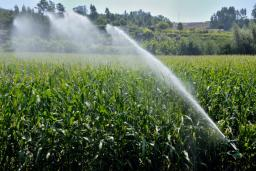We would be pleased if you find an error in the word problem, spelling mistakes, or inaccuracies and send it to us. Thank you!Tips to related online calculators

#### You need to know the following knowledge to solve this word math problem:

We encourage you to watch this tutorial video on this math problem:

## Next similar math problems:

• Center traverseIt is true that the middle traverse bisects the triangle?
• Reference angleFind the reference angle of each angle:
• CosineThe point (8, 6) is on the terminal side of angle θ. cos θ = ?
• LiftThe largest angle at which the lift rises is 16°31'. Give climb angle in permille.
• Circle arcCalculate the area of the circular arc in m2 where the diameter is 290 dm and a central angle is 135°. Result round to three decimal places.
• 10 pieces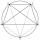How to divide the circle into 10 parts (geometrically)?
• TreeHow tall is the tree that observed in the visual angle of 52°? If I stand 5 m from the tree and eyes are two meters above the ground.
• High wallI have a wall 2m high. I need a 15 degree angle (upward) to second wall 4 meters away. How high must the second wall?
• Circle inscribed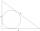Calculate the perimeter and area of a circle inscribed in a triangle measuring 3 , 4 and 5 cm.
• RT - inscribed circleIn a rectangular triangle has sides lengths> a = 30cm, b = 12.5cm. The right angle is at the vertex C. Calculate the radius of the inscribed circle.
• SAS triangleThe triangle has two sides long 7 and 19 and included angle 36°. Calculate area of this triangle.
• Equilateral triangleHow long should be the minimum radius of the circular plate to be cut equilateral triangle with side 19 cm from it?
• Inscribed triangle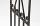To a circle is inscribed triangle so that the it's vertexes divide circle into 3 arcs. The length of the arcs are in the ratio 2:3:7. Determine the interior angles of a triangle.
• Cathethus and the inscribed circleIn a right triangle is given one cathethus long 14 cm and the radius of the inscribed circle of 5 cm. Calculate the area of this right triangle.
• Circle sectorCircular sector with a central angle 80 ° has area 1257 cm2. Calculate its radius r.
• ReflectorCircular reflector throws light cone with a vertex angle 49° and is on 33 m height tower. The axis of the light beam has with the axis of the tower angle 30°. What is the maximum length of the illuminated horizontal plane?
• Equilateral triangle v3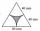Calculate the content of the colored gray part. Equilateral triangle has side length 8 cm. Arc centers are the vertices of a triangle.